# Laws of Cavitation Bubbles

 We consider a cone with height   h    and diameter   d    which is moved horizontally in direction of its axis of symmetry with the velocity  v   through water. Its drag coefficient   cw   is found in the preceding figure. Gaining sufficiently high velocity   flow separates from the base of the cone generating an approximate rotational ellipsoidal cavity the so called "cavitation bubble" with a contour given by the equation : Equation (1)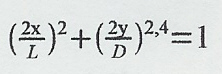The ratio of maximum diameter of the bubble   D   versus its length   L   is given by the relation: Equation (2)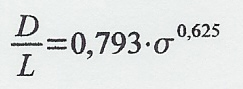where  σ  is defined as: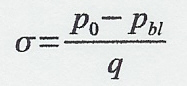with   po     pressure at location of motion          pbl    pressure within the cavitation bubble          q      dynamic pressure = ρ/2*v²          ρ     density of water Pressure   po   at location of movement is given by pressure in depth   T    plus atmospheric pressure on water surface    PL  . Therefore   σ : Equation (3)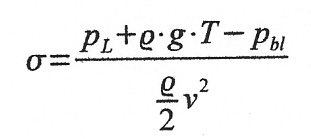with    pL   pressure on the water surface,            T   depth and            g   gravitational acceleration. According to Reichardt the drag of a cone with drag coefficient   cwo   and the base diameter   d  equals the drag of a fictive body with the drag coefficient   σ   related to its maximum cross section area of the cavitation bubble with diameter   D :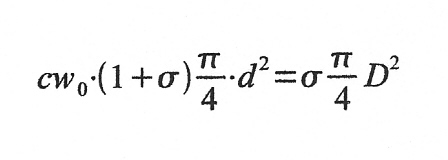By transformation results for the maximum bubble diameter  D   depending on cone diameter   d   , drag coeffcient  cwo    and cavitation number  σ  : Equation (4)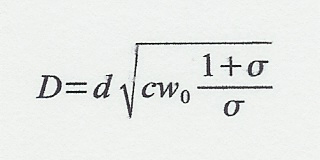The force required to move the cone through water is given by the drag coefficient of the cone (Kegel), the cross section   F  of the cone, the density of water and the velocity   v  . Equation (5)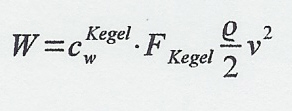The normal force on a cone (Kegel) moving with an angle of attack   α   versus direction of motion is calculated by: Equation (6)with       α               angle of attack              caαocone     coefficient of lift per degree of α (last picture in last chapter) These 6 equations are sufficient for calculation of supercavitating objects. For illustration we calculate an example: A cylinder of diameter 25 cm is axially and horizontally moved through water in a depth of 5 m with velocity 100 m/s. Let us assume a pressure within the bubble of 0 bar then the cylinder produces a cavity with length 15 m and maximum diameter of 1.3 m. The necessary force to move the cylinder would be  2*105 Newton.